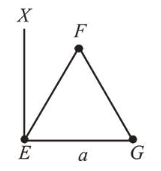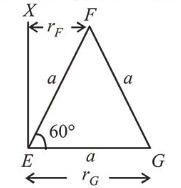# An massless equilateral triangle E F G$of side ' a ' (As shown in figure)Question: An massless equilateral triangle$E F G$of side '$a$' (As shown in figure) has three particles of mass$m$situated at its vertices. The moment of inertia of the system about the line$E X$perpendicular to$E G$in the plane of$E F G$is$\frac{N}{20} m a^{2}$where$N$is an integer. The value of$N$isSolution: (25) Moment of inertia of the system about axis$X E$.$I=I_{E}+I_{F}+I_{G}\Rightarrow I=m\left(r_{E}\right)^{2}+m\left(r_{F}\right)^{2}+m\left(r_{G}\right)^{2}\Rightarrow I=m \times 0^{2}+m\left(\frac{a}{2}\right)^{2}+m a^{2}=\frac{5}{4} m a^{2}=\frac{25}{20} m a^{2}\therefore N=25\$